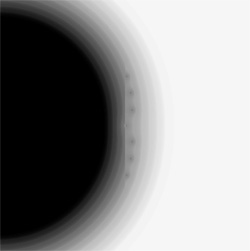www.johngill.net

 Mathematicae Varietas

5

Power Series

Functions analytic in a neighborhood of z = 0, can be expanded as power series (Taylor's Series) there:,

with radius of convergence.

Within the circle of convergence, the series converges uniformly to F(z), and outside the circle, it diverges. The convergence behavior on the circumference of the circle varies with the function.

The following graphics show the regions of convergence of several functions, and how well the truncated series match the various functions, with darker hues indicating best approximations, and light hues, either divergence or poorer approximations.

Example 1n = 10   and  Image radius 4

Example 2n = 50   Center of Image: 1+0i   Image radius  1
Dark points are anomalies slightly outside circle of convergence,
showing better approximations than expected.

Example 3n = 5 (Polynomial of degree 10)    Image Radius 30
Red spots indicate better approximations than usual for that radius -
blue shows poorer approximationsn = 10  (Polynomial of degree 20)     Image Radius 30